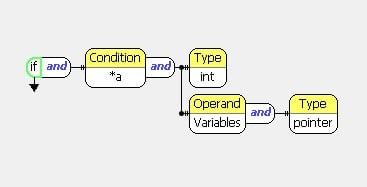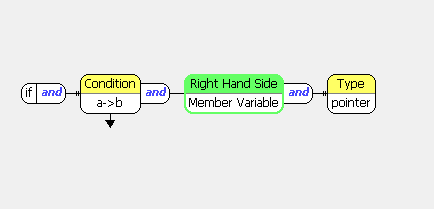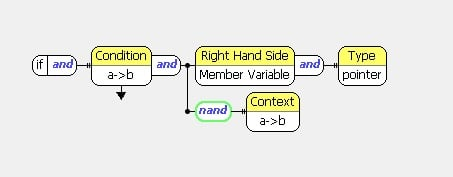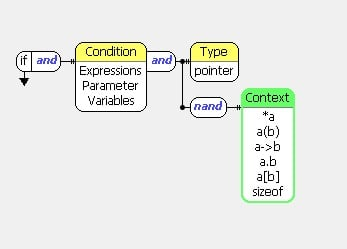Welcome to the new Parasoft forums! We hope you will enjoy the site and try out some of the new features, like sharing an idea you may have for one of our products or following a category.

# How do I create a custom static analysis rule to check that a variable is of a certain data type?

More specifically, how do I check that the variable in an if condition is of a pointer type, when the variable is a pointer dereference? I have tried "left hand side:variable" > "filter:pointer", "left hand side:variable" > "type:pointer" and "left hand side:pointer", and none of them works. This rule wizard is proving to be a waste of money...

Tagged:

• Hi,
You can check in Rule Wiazrd types of variables/parameters/expressions/constants. For every element of code (node) the property 'Type' should be used in Rule Wizard rule. For example:
void f() {
int* p;
if(*p){} // type: p - pointer, type *p - int
}
This 'if' statement is detected by the following rule:I'm note sure if it is the case you want to detect, but for more complicated code would be possible to extend this rule.

• Hi, thanks for helping. My condition is slightly different. It is a property of a struct pointed by a pointer. For example, p->var where p = pointer and var = bool. I'm trying to check that var itself is not a pointer.

• In simple case like this:

```struct S {
int i;
int* sp;
};

void f() {
S* s;
if(s->i){}   // (1) type: s - pointer, type i - int
if(s->sp){}  // (2) type: s - pointer, type sp - pointer
}
```

can be used the rule that detects a->b expression and checks that RHS operand of this expression is a member variable of pointer type.It detects (2) and does not report on (1)
For more complicated cases (for example when instead of 'sp' as RHS operand is used complex expression) this rule can be extended.

• Thanks for the help. Does this work for a->b->...->n as well?

• No, for this case additional modification is needed:This rule reports on (2) and does not report on (1) from the code below:

```struct S1 {
int i;
int* sp;
};

struct S {
S1* s1;
};

void f() {
S* s;
if(s->s1->i){}   // (1) type: s - pointer, type i - int
if(s->s1->sp){}  // (2) type: s - pointer, type sp - pointer
}
```
• Hmm...I don't quite understand what the context node is doing...

• The 'Context' property usually lets you find an outer node.
For example:
a + (b - c); expression (b-c) is in context of expression (a+())
It can be used for functions/types/statements as well, for example:
void f() { a = b; } expression (a = b) is in context of the 'f' function.
Additional information you can find in https://docs.parasoft.com/display/RW/General+Commands

• Thanks for the help so far, but I think the answer may only resolve part of my problem. Let me correct my question in order to give a better idea of the scope of my problem.
How do I find out if the if condition contains a pointer. There is no restrictions to the if condition.
Does the proposed solution also work for the following conditions?
1. if(ptr)
2. if(a.ptr)
3. if(ptr != NULL)
4. if(ptr->ptr != nullptr)
5. An unknown combination of class, struct and pointer ending at a pointer. For example, if(a.b.ptr1->c.ptr2)

Side question: is NULL considered a nullptr constant?

• I mean you want to detect when a pointer is used in 'if' condition, but it is allowed to use an object pointed by this pointer (dereferenced pointer). You can detect parameter/variable/expression of pointer type and exclude use of them in expressions that can change the type.
It can be detected by this rule:It works on the following code:

```struct S1 {
int i;
int* sp;
};

struct S {
S1* s1;
int o;
};

void f(int* ptr) {
S* s;
S so;
if(ptr);         // Violation
if(*ptr);        // Violation
if(ptr == 0);    // Violation
if(0 != ptr);    // Violation
if (so.s1 > 0);  // Violation
if (so.s1->i > 0);  // OK
if (so.s1->sp > 0);                    // Violation
if ( (so.s1->i > 0) && (so.s1 > 0) );  // Violation
if ( (s->o > 0) && (s > 0) );          // Violation
if(s->s1->i){}                         // OK
if(s->s1->sp){}                        // Violation
}
```

If the NULL is a macro, then it is detected as the code after preprocessing (0, (void*)0, nullptr (in C++11) or something else).